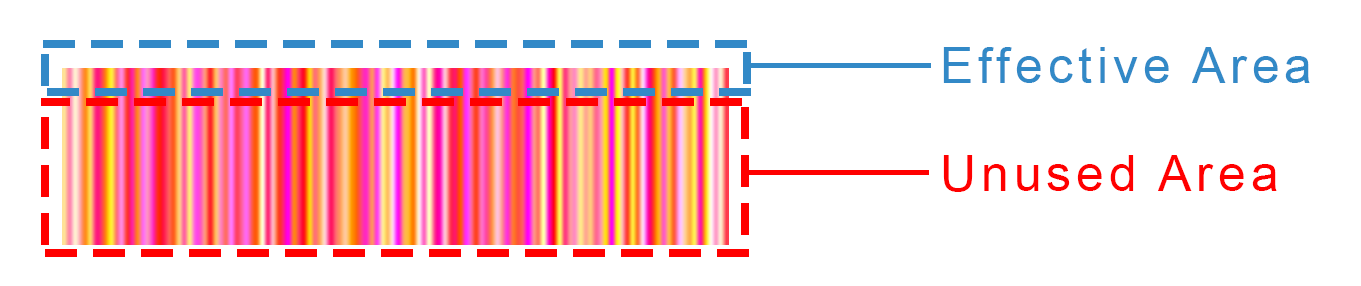# Web Interface Update: Hack the Noise and More

In order to have a more interesting user experience, we introduce some updates to our web interface.

#### For People who Want to Hack the Noise:

With growing interest in playing with and manipulating the noise (here, here, and many more), we here introduce a more direct (and somewhat hackish) way to control over the noise. You can specify the noise by importing your own noise image. The noise image can be of common formats (such as PNG, JPG, etc.), and must be colored, with the resolution of $128 \times 34$ ($128$ columns by $34$ rows). In the process of importing noise, only the first row of the image is used, and the rest rows are ignored, as shown below:The first row, consisting of $128$ pixels, specifies the $128$-length noise vector $v$. The $i$-th element of the noise vector ($v_i$) is calculated using

with $G_{i}$ and $B_{i}$ are the values of the G channel and the B channel of the $i$-th pixel respectively. The R channel (and the A channel, if exists) is/are ignored.

PS: A noise vector with elements near $0$ usually generates good images.